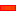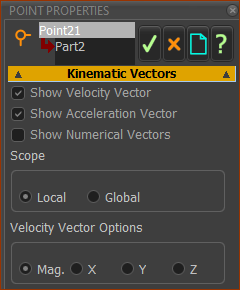﻿ Dialog: Point: Vectors and Point Location

# Dialog: Point Position & Vectors

## Point Position & Vectors dialog-box

Use this dialog to:

 • Show Velocity and Acceleration Vectors of a Point as you cycle the model.
 • Find the exact position of a Point on the Mechanism-Plane
 • Find the position of a Point on the Part-Plane, using Part Coordinates.
 • Edit the position of a Free-Point that does not have constraints added with the Part-Editor, using Part Coordinates

You do not need to open the Part-Editor to edit the position of a Point with this dialog

Note: With this dialog, you can edit the Point's position ONLY when it does not have any geometric constraint added to it.

### The Point Position and Vectors dialog-box

Edit a Point element to open the Point Position and Vectors dialog.There are three separators:

 • Show or Hide VectorsVelocity and Acceleration Vector check-boxes

If you show Velocity or Acceleration vectors, or both:

 • Each vector shows only when the Point is moving relative to the Mechanism-Plane
 • The length of each vector is proportional to the Point's instantaneous Velocity and Acceleration.
 • The magnitude of each vector shows at the arrowhead of each vector
 • The colour [default] of the vectors are Velocity, Acceleration
 • Each vector updates its value and length each machine step [see Machine Settings dialog-box > Cycling Parameters > Number of Steps]

Scope

You can display the vectors relative to the:

 • Local xyz axes - Mechanism Axes [default]
 • Global XYZ axes - MODEL AXES.

Velocity Vector Options

The Velocity vectors can show as one of::

 • Magnitude of the Velocity [default]
 • X component of the motion
 • Y component of the motionTo change the scale and length of the vectors, use the buttons in the Feedback-Area:

 • Click the up or down buttons below 'Vel' to edit the Velocity Vector
 • Click the up or down button below 'Accel' to edit the Acceleration VectorMechanism Coordinates [Read-Only]The Point coordinates [X, Y, Z ] relative to the Origin of the Mechanism-Plane (and Base-Part). The X, Y, Z dimensions are Read-Only.The  Origin [0,0, 0] of the Mechanism-Plane is the intersection of the small X- and Y-axes in the Base-Part.The Point coordinates [x,y] are relative to the Part's Origin.

 • Its Origin  is the start-Point of the CAD-Line
 • Its X-axis is from the start-Point of the CAD-Line to its end-Point.

Lock and Edit Point's Position.

 ◉ Edit in Part-Editor

The Point remains 'free' and you can edit it in the Part-Editor.◉ Lock and Edit here
 • Use the x and y boxes to edit the x, y coordinates of the Point with Point coordinates

With this option

 • You will not be able to edit it in the Part-Editor.

To use this option:

 • The Point must be free of constraints and dimensions in the Part-Editor

Tutorial and Reference Help Files for MechDesigner and MotionDesigner 13.2 + © Machine, Mechanism, Motion and Cam Design Software by PSMotion Ltd